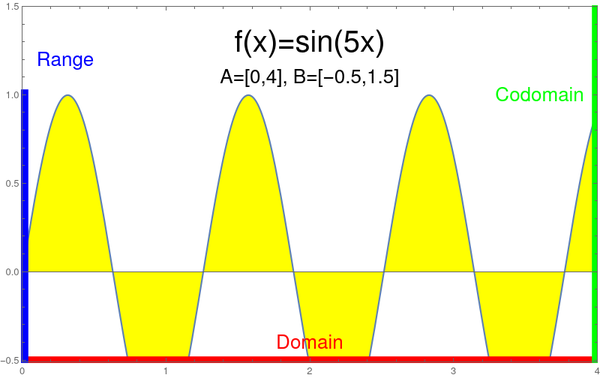# Questions

If a question does not appear here, don't hesitate to contact Oliver. If it is asked frequently or of potentially more general interest, we will add it here.

• Why do we look at domain and codomain? : this is often not stressed in calculus especially in older texts but the modern point of view is that functions always come with a domain A on which we consider the function and a codomain where we look at it. Typically the function should take values in the codomain. Think of A and B as the intervals defining the rectangle, on which you look at the function. The need to specify where one considers the functions came with computer science. The domain should be part of the domain of definition, where the function is defined. The range is the part of the codomain which is reached by the function. Graphs can be assigned a domain and codomain. Here is an example in Mathematica which explains this: we plot a function on the domain [0,4] and codomain [-0.5,1.5]. The range is the interval [-0.5,1].
```      Plot[Sin[5 x], {x, 0, 4}, PlotRange -> {{0, 4}, {-0.5, 1.5}}]
```• What is an hourly? Answer: Oliver is a dinosaur teaching here already since 2000 and uses the name which was then still used for midterm exams. Harvard has its unique jargon and ``hourly" is one of the forgotten ones (since exams are mostly longer than an hour these days).
• What are the prerequisites? Answer: You need a solid precalculus knowledge including exposure to trigonometric functions. There are lots of resources online. An example.
• Is there an option to see the course at a different time. Answer: since we have a single section course in the spring, you need to be able to attend the class during the regular class time.